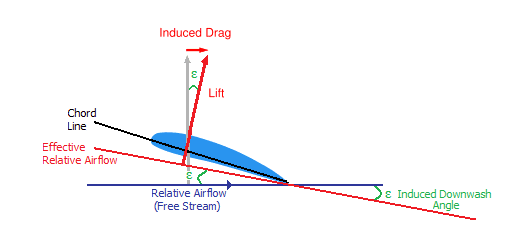# Induced Drag Airfoil

GlynnHeeswijk
Induced drag of an airfoil should be inversely proportional to velocity^2 wiki states "Since induced drag is inversely proportional to the square of the airspeed" Ok but [Induced Drag = 0.5 * Density * Velocity2 * Wing Area * Induced Drag Coefficient] which is proportional to the veloicty^2 and not inversely proportional and the term CDi which is CDi = (k*CL2)/ (pi*AR) does not have a velocity term thus how can it be inversely proportional?

Last edited:

Homework Helper
Induced drag is normally defined as sin(θ) x lift, where θ = effective angle of attack, or θ = effective angle of deflection of air flow relative to the aircraft, which is what the wiki article diagram shows.As airspeed increased, the angle of diversion decreases, so that sin(θ) x lift decreases with speed^2. This is an approximation that starts to deviate from reality once above mach 0.3->0.4, depending on the aircraft.

The wiki article claims that the source of induce drag and downwash (lift) is related to wingtip vortices:

wiki_Source_of_induced_drag.html

However other web sites point out the somewhat obvious fact that induced drag on an 747 at takeoff and landing (slow speed, high amount of induced drag) would rip the wing tips apart if the source of induced drag was solely due to wingtip vortices or wingtip and trailing edge vortices (some airfoils minimize trailing edge vortices for a given load and speed range).

Last edited:
GlynnHeeswijk
But CDi = (k*CL2)/ (pi*AR) (coefficent of induced drag) doesn't have a velocity term and neither does CL (coefficent of lift) so I dont see why it would change with velocity.

Homework Helper
But CDi = (k*CL2)/ (pi*AR) (coefficent of induced drag) doesn't have a velocity term and neither does CL (coefficent of lift) so I dont see why it would change with velocity.
In level flight, the amount of lift generated is constant, so CL and CDi would decrease with speed^2, a reasonable approximation until you reach speeds above mach 0.3 or 0.4, where a much more complex form of mathematical model is requried.

GlynnHeeswijk
In level flight, the amount of lift generated is constant, so CL and CDi would decrease with speed^2, a reasonable approximation until you reach speeds above mach 0.3 or 0.4, where a much more complex form of mathematical model is requried.

That kinda makes sense as you would lower angle of attack to keep the lift constant as velocity increases thus lowering the lift coefficient which would in turn lower the induced drag coefficient.

Homework Helper
That kinda makes sense as you would lower angle of attack to keep the lift constant as velocity increases thus lowering the lift coefficient which would in turn lower the induced drag coefficient.
It lowers the induced drag coefficient, but increases the parasitic drag coefficient. I'm not sure why there is such a focus on separating total drag into induced and parasitic components. In the real world, a wing design is a compromise between lift/(total drag) ratio for a given speed range and cost to manufacture.